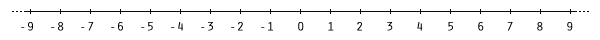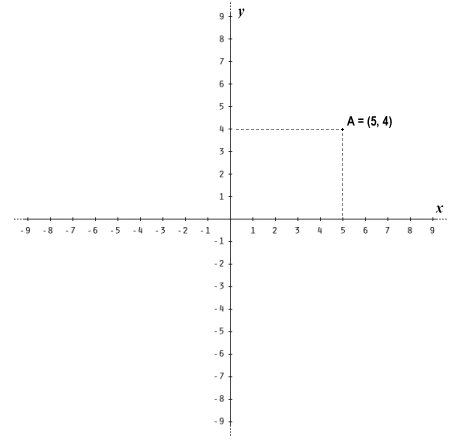# Lines, Rays and the Coordinate System

In order to create a coordinate system that allows us to specify the location of a point on a two-dimensional plane (relative to the origin), we need to make some decisions. If we visualise the plane as a flat piece of paper that stretches away from the origin in every direction to infinity, we can choose some arbitrary direction and imagine a line that extends from the origin to infinity in that direction. We will even mark the line out using a pencil as far as we feel is necessary to ensure that we remember where it is. We will then extend that line in the opposite direction, still using our pencil, so that whichever direction we are facing we can still get our bearings. Just like a point, however, a line has no physical existence. In fact, we can think of a line as the collection of all of the points that exist between the origin and infinity in a particular direction, plus all of the points that exist between the origin and infinity in the opposite direction. The line is not dimensionless, because it has infinite length. It has no breadth or depth, however, and is therefore one-dimensional. The line we drew with the pencil is not really a line, but it serves well enough as a marker to tell us where the line is, in the same way that a dot represents the position of a point.

If we now establish some arbitrary unit of measurement, we can mark the line at equidistant intervals so that we can tell how far in one direction or the other a given point on the line is from the origin. Since the line itself stretches to infinity in both directions, we can't very well mark these intervals all the way along it from one end to the other. We will mark them for as far in each direction as suits our purposes, and leave it at that. Being clever, we have decided to label the origin as zero (0), and all the intervals in one direction we will label incrementally, starting with one (1). We will label all the intervals in the other direction incrementally too, but this time we will use negative numbers starting with minus one (-1). At this point in time, our newly created geometric universe looks like this:A one-dimensional coordinate system

Not particularly impressive, but it's a start. We still have a problem however, because unless the points we create are actually on the line, their exact position on the two dimensional plane cannot be specified. We could of course say that a particular point is on one side or the other of the line, close to a particular interval marker. This is not very helpful though, because there is nothing to differentiate one side of the line from the other, and anyway we still don't know exactly how far away the point is from the line. There is a clue there, however, as to what we need to do. We queried how far a point was "away from the line". That depends, of course, on which point on the line we measure the distance from. Being fairly logical people, however, we will use the shortest distance between the line and our point.

Let's assume we have created some arbitrary point not too far away from our line. If we trace the shortest possible path between the point and the nearest point to it on our line using a pencil, we might notice something interesting. We call this path a line segment because it has a finite length. By definition, a line stretches to infinity in both directions. A line segment is any part of a line that connects two designated points on the line (while we're on the subject, we can think of a line as being made up of an infinite number of points). When we talk about drawing lines, in the context of a diagram or a geometric construction, we should probably talk instead about drawing line segments. That would be somewhat pedantic, however, and the context should be sufficient to allow what we say to be correctly interpreted. Anyway, to get back to the line segment we have drawn between our newly created point and the line, what can we say about it?

If we stand on the line where the line segment joins it, and look in the direction of our point, we should notice that in order to turn and face directly along our line in one direction or the other requires the same degree of movement. In other words, the line segment does not slant towards one end of the line or the other. Remembering that we don't know about angles yet, we will make a note of this for future reference, but it seems like a useful thing to be aware of. In fact, let's create another point, this time making sure that the new point is closer to the origin than any other point on the line. We will now construct a second line that intersects both our new point and the origin. By definition, it will extend to infinity in either direction. Again, we will draw the line on a sheet of paper in both directions as far as is practical, and mark off intervals on it using the same units we used for the first line. Now our geometric universe looks like this:A two-dimensional coordinate system

We again made an arbitrary decision on numbering the intervals - positive numbers in one direction (starting with one, of course) and negative numbers in the opposite direction, starting with minus one. The only problem now is that both of these lines look exactly the same. We need to be able to differentiate between them in some way. Let's assume we can float above the plane and look down on it. We will orient ourselves so that our head is pointing along one of the lines in the positive direction, and we can extend our right arm so that it points along the other line, also in the positive direction. Let's label these lines so that the line along which we are pointing with our right hand will be labelled x, and the line along which our head is pointing will be labelled y. Now, from our point of view, positive values of x appear to be on the right-hand side of the origin, and negative values of x to the left. Similarly, positive values of y are above the origin, and negative values are below.We now have a horizontal x-axis and a vertical y-axis

Of course, the use of the terms left and right, up and down are used purely for convenience, so that we can orient ourselves in our new two-dimensional universe from a human perspective. If you are looking at the illustration above on a computer screen, the chances are that the y-axis will indeed be (more or less) vertical. If you were to see the same illustration in a book lying open on your desk, it would in fact be lying in a horizontal plane. Interestingly, however, in both cases your perception is probably that of a vertical line. This is simply because of the way we have learned to look at such things. At least we now have a frame of reference within which we can specify the exact location of a point. We do this in terms of its horizontal distance to the left or right of the y-axis and its vertical distance above or below the x-axis.

The meaning of the intervals marked on each line will depend on what the points we are drawing represent. If we are creating points that define the boundary of a parcel of land, the distance between each interval could represent yards or metres, or some multiple thereof. If the boundary in question was a county boundary or the border of some state, the intervals could represent miles or kilometres, or even larger units of measurement. On the other hand, we could be specifying the position of components on a circuit board, in which case the intervals are more likely to represent millimetres or fractions of an inch. Whatever the relative scale of the objects we wish to represent, we now have the means to determine the position of any point on the plane relative to the origin, and indeed relative to other points on the plane.

The points we create can now be specified in terms of their Cartesian coordinates. The set of coordinates that defines a point consists of two numbers. The first number tells us how far away the point is from the y-axis in the horizontal direction. We can determine this distance by drawing (or imagining) a vertical line segment that connects the point to the x-axis. The distance between the origin and the point where the line segment meets the x-axis gives us the value of the first number in our coordinate pair. The second number tells us how far away the point is from the x-axis in the vertical direction. We can determine this distance by drawing (or imagining) a horizontal line segment that connects the point to the y-axis. The distance between the origin and the point where the horizontal line segment meets the y-axis gives us the value of the second number in our coordinate pair. Consider the following illustration:Point A has xy coordinates of (5, 4)

Note that it is a common convention in geometry to label points using upper-case characters (lines and line segments are frequently labelled using lower-case characters). Point A is a horizontal distance of five units from the y-axis and a vertical distance of four units from the x-axis. It is said to have xy (or Cartesian) coordinates of (5, 4). In other words, the x coordinate is four, and the y coordinate is five. If a point is to the left of the y-axis, the value of its x coordinate will be a negative number. Similarly, if a point is below the x-axis, its y coordinate will also be a negative number. If we have two points on a plane, we can draw a line segment that connects them. If we have three points on a plane (providing they do not all fall on the same line), we can connect each point to each of the other two points using line segments, and we will have a triangle. In fact, we can draw lots of different types of two dimensional objects just using points and line segments.

So far we have not mentioned rays. A ray is different from both a line and a line segment. Imagine some arbitrary line on our plane. A line segment belonging to that line is a part of the line that extends between any two designated points on the line (let's call them A and B). The line segment has a starting point and an ending point, and is thus finite in length. The line itself also goes through the same two points, but extends beyond them in both directions to infinity. It has no starting or ending point. A ray is neither a line nor a line segment. It has a starting point (let's assume this is A) and may well pass through B, but will then continue on to infinity. Rays are useful when we want to talk about angles, which we will be doing on another page.